Let’s talk about activation function in artificial neural network and some questions related of it.

# Activation Function

Activation function of a node defines the output of that node given an input or set of inputs. It decide whether the node will be “ON” (1) or “OFF” (0) depending on input. However, only nonlinear activation functions allow such networks to compute nontrivial problems using only a small number of nodes. In artificial neural networks, this function is also called the transfer function. - Wikipedia

As it is described in the equation (1), artificial neural network calculates a weighted sum of input and adds a bias. However, since the range of value $Y$ is between $-\infty$ and $+\infty$, the neuron does not know the bounds of the value and when to “ON” or “OFF” the neuron itself. This is why we use activation function to make a bound of the value $Y$ and decide whether it should be “ON” (1) (activated) or “OFF” (0) (deactivated). (The idea of neuron to be activated or deactivated is from how our brain and neuron on the brain works.)

## Binary Step FunctionThe easiest activation function we can think of is binary step function. The neuron can be activated (1) if $Y \ge 0$, deactivated (0) otherwise. This kind of activation function will work for binary classification problem which has to decide 1 or 0. However, if you have to classify more than two classes, this would not a good choice to use. For example, if you have classify four classes, class1, class2, class3, class4, and it gives you 1 for class1, class2 and 0 for class3, class4, what would you choose for the result? As the output 1 indicates 100% activated, it is hard to choose between class1 and class2 with valid reason. Therefore, we rather need to get probabilistic activation output such as, 50% activated and 70% activated. Alternatively, we need strength or probabilistic activation values rather than just binary activated or deactivated.

## Identity and Linear Function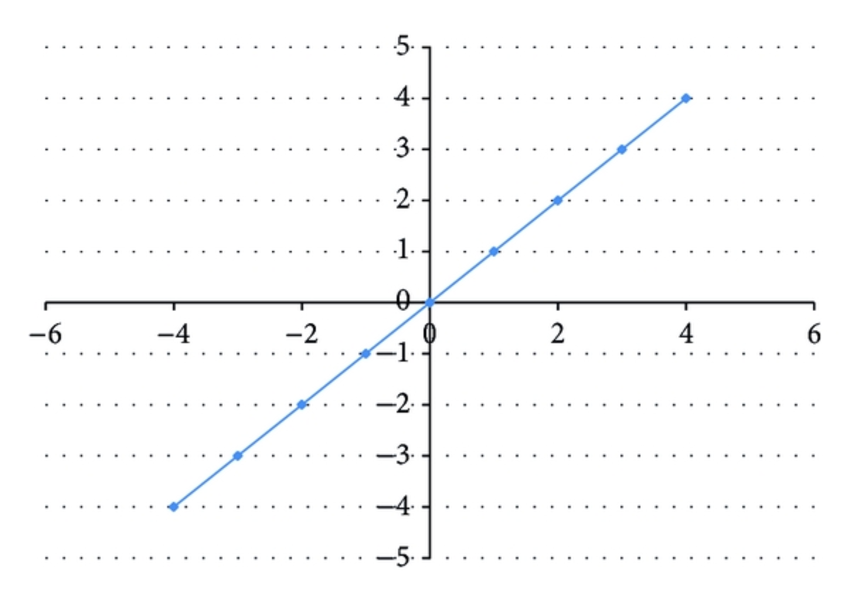Linear function or identity function is the first thing we can try to use to handle the problem of binary step function. Using linear function makes the output from the activation function proportional to input and this gives a range of activations which is not binary. So, if there are more than one neuron is “ON”, we can choose one based on taking the max or softmax.

However, using linear function as activation function has few problems. First, no matter how many layers we stack on neural network, if all are linear function for each layer, the final activation function of the last layer will be just a linear function of the input of first layer. It means all the layers we stacked can be replaced by a single layer and it will make stacking layers meaningless.

Second, as the derivative of equation (2) with respect to $x$ is $c$, this gradient gives no relationship with $x$ when we use gradient descent algorithm for training. Even though we have an error in prediction, the changes made by back propagation will be constant and it does not depend on the change in input $\delta(x)$. This is a huge problem for training a network since the network need to be trained based on the input and its error in prediction, not constant value.

## Sigmoid Function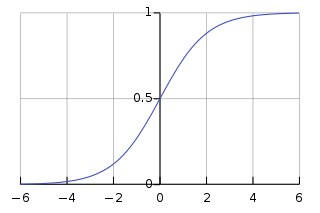Sigmoid function is well-known activation function in classification problem. As you can see on the figure, it looks like step function, but has smooth curve which will give us analogue activation unlike step function. So it can be used for multi-classes classification problem. Moreover, as it is nonlinear function, combination of sigmoid function will be nonlinear as well and so we can stack layers.

This function has tendency that it brings the $f(x)$ values to either end of the curve. The $x$ values between -2 and 2 have very steep $f(x)$ values, which mean, small changes of $x$ values will make big changes on $f(x)$ values. This property gives advantage on classification problem because it brings the activations to either side of the curve (above $x=2$ and below $x=-2$) and makes clear distinctions on prediction. One more advantage of the sigmoid function is that the output range of it is $(0,1)$ compared to its of linear function $(-\infty,+\infty)$. It gives valid activation bounds and prevent the output exceeds the limit to calculate.

One drawback of sigmoid function is that it can cause “gradient vanishing problem”. On the either end of the sigmoid function (above $x=4$ and below $x=-4$), $f(x)$ values change very slowly by $x$ values and the gradient in this region will be small. Thus, gradient cannot make enough change because of extremely small value and it can be vanished. This problem will cause significantly slow training or refuse to learn further.

The other drawback is that sigmoid outputs are not zero-centered. This could cause undesirable zig-zagging dynamics in the gradient updates for the weight, because if the data coming into a neuron is always positive, then the gradient on the weights will become either all be positive or all negative during backpropagation.

There has been several tricks introduced to avoid this problem, but it has recently fallen out of favor and rarely used.

## Hyperbolic Tangent (Tanh)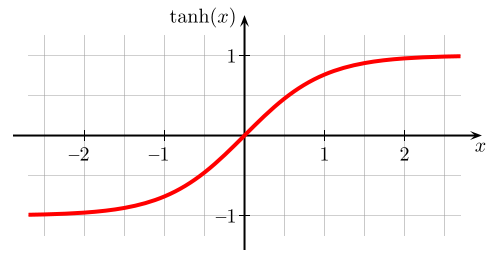The tanh function is a scaled sigmoid function as shown in the equation (3) and (4). I has very similar look with sigmoid function, has bound range between [-1, 1] and zero-centered. As it has non-linearity, we can stack layers, and as it has bound range between [-1, 1], the activation would not blow up. Furthermore, the tanh function is always perferred to the sigmoid function, because it is zero-centered.

## Rectified Linear Unit (ReLU)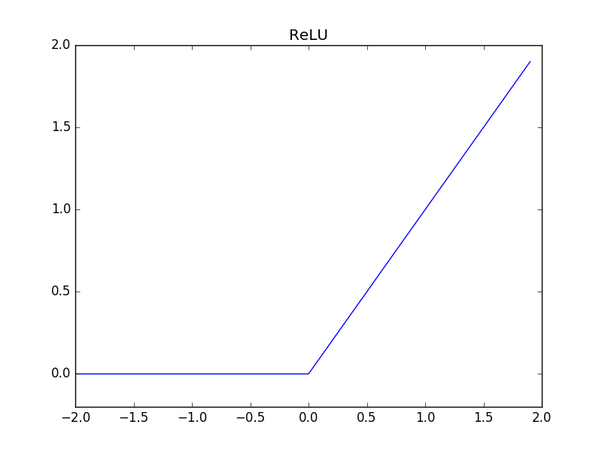ReLU has become a very popular activation function these days. It outpus x if x is positive and 0 otherwise. ReLU looks like to have the problem of linear function, but it is nonlinear and the combination of ReLU is also nonlinear. (As it is a good approximator, any function can be approximated with combinations of ReLU) And the range of ReLU is [0, inf), so it can blow up the activation.

First advantage of ReLU is that it greatly accelerate the convergence of stochastic gradient descent compared to sigmoid and tanh functions. This is because of its linear and non-saturating form. (‘Saturate’ means is that the function plateaus out or saturates beyond some range. For example, $tanh(x)$ saturates on [-1, +1] but ReLU does not at least on the positive side) Second advantage is that it can be implemented by simply thresholding a matrix of activations at zero, when tanh and sigmoid neurons involve expensive operations such as exponentials.

However, it has disadvantage of “dying ReLU problem” which means ReLU units can be fragile during training and can die. This is because the gradient can go towards zero for negative $x$ and it will make the units go into that state will stop being trained to variations in error and input. This problem usually happens if the learning rate is set too high but with proper setting of the learning rate, the issue will be less.

## Leaky ReLU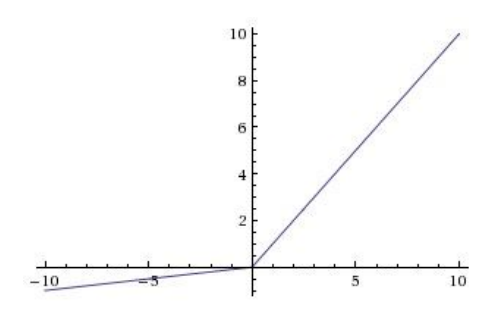There are variations in ReLU to handle the dying ReLU problem and Leaky ReLU is one of them. It simply makes the horizontal line of ReLU into non-horizontal component as shown in equation (5). The main idea of it is to let the gradient be non-zero and recover during training eventually. However, the consistency of the benefit using this function is not clear yet.

## Maxout

Other types of units have been proposed that do not have the functional form as equation (1). One popular form is the Maxout neuron that generalizes the ReLU and leaky ReLU function. Therefore, maxout neuron have all the advantage of ReLU unit while not having dying ReLU problem. However, it doubles the number of parameters for every single neuron which lead to a high total number of parameters.

## Softmax

The softmax function is a generalization of the logistic function that squashes a $J$-dimensional vector $x$ of arbitrary real values to a $J$-dimensional vector $f_i(\vec{x})$ of real values in the range [0,1] that add up to 1. In probability theory, the output of the softmax function can be used to represent a categorical distribution which is a probability distribution over $J$ different possible outcomes. Therefore, it is used in multiple classification logistic regression model by giving probability output for each class. And it is used in different layer level, not in unit level.

## Other Activation Functions

• Activation functions of one fold x from the previous layer or layers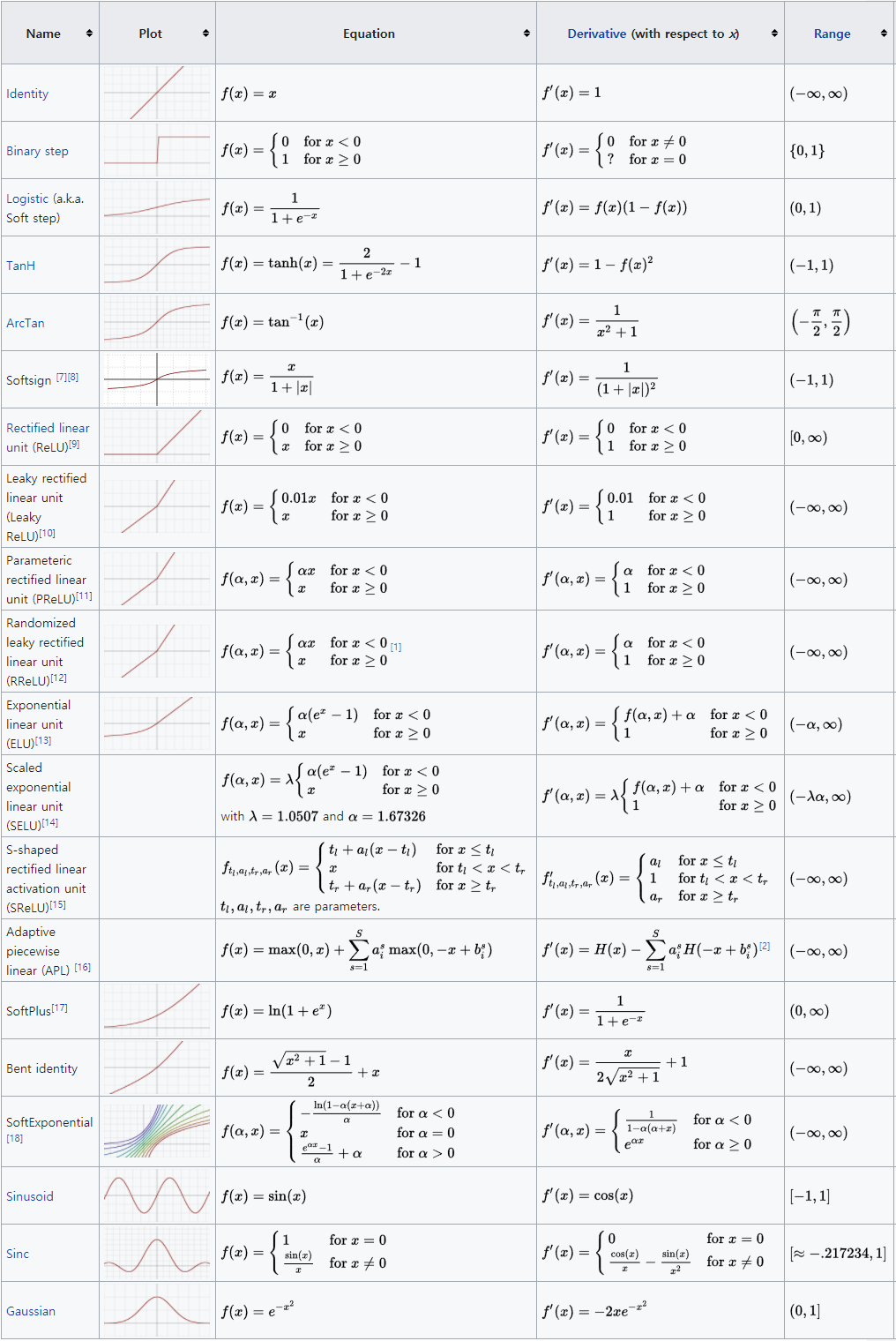• Activation functions of not one fold x from the previous layer or layers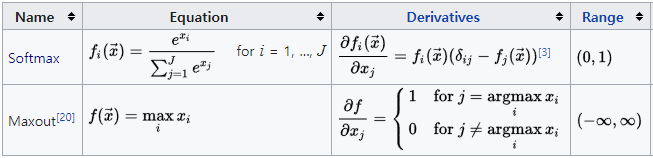# How and what to use?

When you start modeling a network, the ReLU non-linearity function is recommended to use, but you have to be careful with learning rates and dying ReLU problem. If you see some dead units or worried of it, try to use Leaky ReLU or Maxout. You do not want to use Sigmoid function, try tanh instead, but it would work worse than ReLU and Maxout. Moreover, it is not common to mix and match different types of neurons in the same network, even though there is no fundamental problem identified.

• Activation function in Wikipedia [Link]